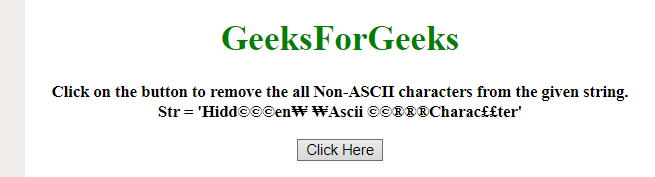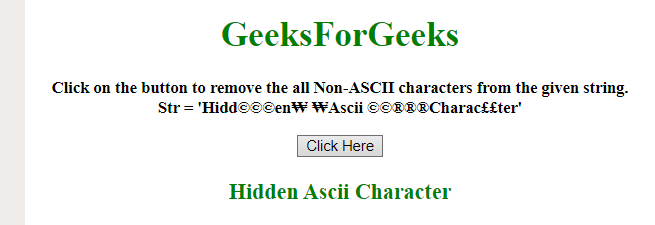Related Articles
How to remove all Non-ASCII characters from the string using JavaScript ?
• Last Updated : 13 Dec, 2019

Given a string containing some Non-ASCII characters and the task is to remove all Non-ASCII characters from the given string. There are two methods to solve this problem which are discussed below:

Approach 1:

• This approach uses a Regular Expression to remove the Non-ASCII characters from the string.
• Only characters that has value from zero to 127 are valid. (0x7F is 127 in hex).
• Use .replace() method to replace the Non-ASCII characters with the empty string.

Example: This example implements the above approach.

 ` ``<``html``> `` ` `<``head``> ``    ``<``title``> ``        ``How to remove all Non-ASCII characters``        ``from the string using JavaScript ?``    ```` `` ` `<``body` `style` `= ``"text-align:center;"``> ``     ` `    ``<``h1` `style` `= ``"color:green;"` `> ``        ``GeeksForGeeks ``    ````     ` `    ``<``p` `id` `= ``"GFG_UP"` `style` `=``        ``"font-size: 15px; font-weight: bold;"``>``    ````     ` `    ``<``button` `onclick` `= ``"gfg_Run()"``> ``        ``Click Here``    ````     ` `    ``<``p` `id` `= ``"GFG_DOWN"` `style = "color:green;``        ``font-size: 20px; font-weight: bold;">``    ````     ` `    ``<``script``>``        ``var el_up = document.getElementById("GFG_UP");``        ``var el_down = document.getElementById("GFG_DOWN");``        ``var str="Hidd©©©en??Ascii ©©®®®Charac££ter";``         ` `        ``el_up.innerHTML = "Click on the button to remove"``                ``+ " the all Non-ASCII characters from the"``                ``+ " given string.<``br``>Str = '" + str + "'";``         ` `        ``function gfg_Run() {``            ``str = str.replace(/[^\x00-\x7F]/g, "");``            ``el_down.innerHTML = str;``        ``} ``    `` `` `` ` ``

Output:

• Before clicking the button:• After clicking the button:Approach 2:

• This approach uses a Regular Expression to remove the Non-ASCII characters from the string like the previous example.
• It specifies the Unicode for the characters to remove. The range of characters between (0080 – FFFF) are removed.
• Use .replace() method to replace the Non-ASCII characters with the empty string.

Example: This example implements the above approach.

 ` ``<``html``> `` ` `<``head``> ``    ``<``title``> ``        ``How to remove all Non-ASCII characters``        ``from the string using JavaScript ?``    ```` `` ` `<``body` `style` `= ``"text-align:center;"``> ``     ` `    ``<``h1` `style` `= ``"color:green;"` `> ``        ``GeeksForGeeks ``    ````     ` `    ``<``p` `id` `= ``"GFG_UP"` `style` `=``        ``"font-size: 15px; font-weight: bold;"``>``    ````     ` `    ``<``button` `onclick` `= ``"gfg_Run()"``> ``        ``Click Here``    ````     ` `    ``<``p` `id` `= ``"GFG_DOWN"` `style = "color:green; ``        ``font-size: 20px; font-weight: bold;">``    ````     ` `    ``<``script``>``        ``var el_up = document.getElementById("GFG_UP");``        ``var el_down = document.getElementById("GFG_DOWN");``        ``var str="Hidd©©©en??Ascii ©©®®®Charac££ter";``     ` `        ``el_up.innerHTML = "Click on the button to remove"``                ``+ " the all Non-ASCII characters from the"``                ``+ " given string.<``br``>Str = '" + str + "'";``     ` `        ``function gfg_Run() {``            ``str = str.replace(/[\u{0080}-\u{FFFF}]/gu, "");``            ``el_down.innerHTML = str;``        ``} ``    `` `` `` ` ``

Output:

• Before clicking on the button:• After clicking on the button:My Personal Notes arrow_drop_up